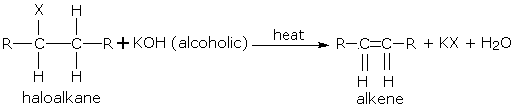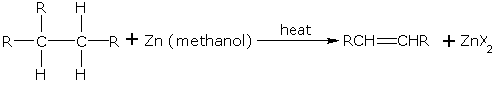# Alkenes from Dehydrohalogenation of Haloalkanes

$$\newcommand{\vecs}{\overset { \rightharpoonup} {\mathbf{#1}} }$$ $$\newcommand{\vecd}{\overset{-\!-\!\rightharpoonup}{\vphantom{a}\smash {#1}}}$$$$\newcommand{\id}{\mathrm{id}}$$ $$\newcommand{\Span}{\mathrm{span}}$$ $$\newcommand{\kernel}{\mathrm{null}\,}$$ $$\newcommand{\range}{\mathrm{range}\,}$$ $$\newcommand{\RealPart}{\mathrm{Re}}$$ $$\newcommand{\ImaginaryPart}{\mathrm{Im}}$$ $$\newcommand{\Argument}{\mathrm{Arg}}$$ $$\newcommand{\norm}{\| #1 \|}$$ $$\newcommand{\inner}{\langle #1, #2 \rangle}$$ $$\newcommand{\Span}{\mathrm{span}}$$ $$\newcommand{\id}{\mathrm{id}}$$ $$\newcommand{\Span}{\mathrm{span}}$$ $$\newcommand{\kernel}{\mathrm{null}\,}$$ $$\newcommand{\range}{\mathrm{range}\,}$$ $$\newcommand{\RealPart}{\mathrm{Re}}$$ $$\newcommand{\ImaginaryPart}{\mathrm{Im}}$$ $$\newcommand{\Argument}{\mathrm{Arg}}$$ $$\newcommand{\norm}{\| #1 \|}$$ $$\newcommand{\inner}{\langle #1, #2 \rangle}$$ $$\newcommand{\Span}{\mathrm{span}}$$$$\newcommand{\AA}{\unicode[.8,0]{x212B}}$$

Alkenes can be obtained from haloalkanes (alkyl halides). These haloalkanes are usually bromo and iodo and less commonly, chloro derivatives. Haloalkanes on heating with alcoholic $$KOH$$ loses one molecule of hydrogen halide to give alkene.##### Example $$\PageIndex{1}$$##### Example $$\PageIndex{2}$$If two alkenes may be formed due to dehydrohalogenation of a haloalkane, the one which is most substituted is the main product.

##### Example $$\PageIndex{3}$$

For example, dehydrohalogenation of 2-bromobutane gives,The order of reactivity of haloalkanes in dehydrohalogenation is, Tertiary > Secondary > Primary. Note: Reactions in which a small molecule like $$H_2O$$ or $$HX$$ is lost are known as elimination reactions.

## Alkenes from Vicinal Dihaloalkanes

Vicinal dihaloalkanes are those dihalogen derivatives of alkanes in which two halogen atoms are on the adjacent carbon atoms. Alkenes can be obtained from vicinal dihaloalkanes by dehalogenation. When such a dihaloalkane is heated with zinc in methanol, an alkene is formed.##### Example $$\PageIndex{4}$$

1,2-dibromoethane gives 1,2-dibromoethane ethene## Contributors

Alkenes from Dehydrohalogenation of Haloalkanes is shared under a CC BY-NC-SA 4.0 license and was authored, remixed, and/or curated by LibreTexts.